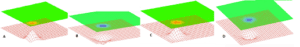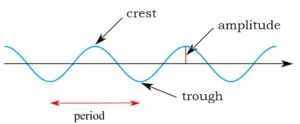Home » absolute square of a complex number

# absolute square of a complex number

« Back to Glossary Index

The absolute square of a complex number is calculated by multiplying it by its conjugate. (The absolute square is not the same as the square of a real number nor the absolute value of a complex number).

Using the example of the complex number 2 + 3i, the absolute square is written |(2 + 3i)|2. Here’s how to calculate the absolute square of 2 + 3i:

(2 + 3i)(2 – 3i) =  4 + 3i(2) – 3i(2) +(3i)(-3i) = 4 +6i -6i -9i2 = 4 + 0 + 9 = 13.  So, the absolute square of 2 + 3i is 13.

Here’s the shortcut for calculating the same absolute square: |(2 + 3i)|2= 22 + 32 = 4 + 9 = 13.

Here’s the general statement of how to calculate the absolute square of a complex number:

|(a + bi)|2 = (a + bi)(a – bi) or a2 + b2

The importance of the absolute square of complex numbers in quantum mechanics.

The absolute square of complex numbers comes up in quantum mechanics. Specifically, the Schrodinger Equation often expresses quantum wave amplitudes using complex numbers. The absolute square of a complex wave amplitude represents the probability of detecting the particle. More precising, it’s the probability of detecting the particle property, such as position, described by the Schrodinger Equation.A localized 3-D wave travels through a quantum field. [Image source: stills from Fermilab video by Dr. Don Lincoln, “Quantum Field Theory” (in the public domain) Jan. 14, 2016; https://www.youtube.com/watch?v=FBeALt3rxEA&feature=youtu.be.]The sequence of diagrams A, B, C, D shows a wave in a quantum field (red netting) moving from the lower left corner towards the center of the field. An associated particle has been detected. It is shown by the orange and blue circles—orange when the wave is spreading upward and blue when the wave is spreading downward. The particle is most likely to be detected at the highest amplitude (height) of the wave.Amplitude of a wave [Image source: modification of Kraaiennest – CC BY-SA 3.0, https://commons.wikimedia.org/w/index.php?curid=4038226. Retrieved Sept. 21, 2017.]Amplitude of a wave. [Image source: Kraaiennest – CC BY-SA 3.0, https://commons.wikimedia.org/w/index.php?curid=4038226. Retrieved Sept. 21, 2017.]

Typically, the amplitude of a quantum wave is expressed as a complex number. The probability of detecting the associated particle is precisely described as the square of the complex number which represents the wave amplitude.

*A complex number is a number which has both a real part and an imaginary part. In the complex number 2 +3i, the real part is 2 and the imaginary part is 3i. The “imaginary part” of a number is a multiple of i, for example, 3i. The symbol i means the square root of -1.

Here is why i is called “imaginary.” The square root of x, when multiplied by itself yields x. However, no number when multiplied by itself yields a negative number such as -1. Thus, i, the square root of -1 is called “imaginary.” In our universe no physical thing can be described as being in the amount -1 or i. Nevertheless, mathematicians and scientists have found many uses for i in mathematical equations. In particular, quantum physics relies heavily on i in its equations.

**If the complex number is (a + bi), then its complex conjugate is (a – bi).

This site uses Akismet to reduce spam. Learn how your comment data is processed.